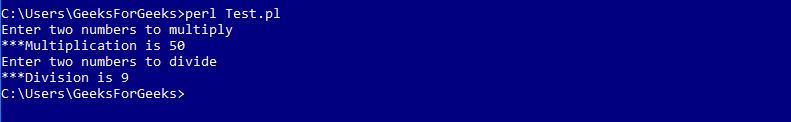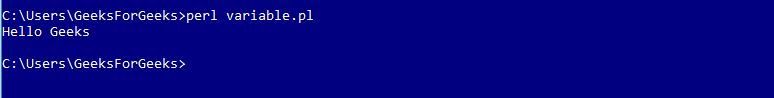# Perl | Modules

• Last Updated : 17 Feb, 2019

A module in Perl is a collection of related subroutines and variables that perform a set of programming tasks. Perl Modules are reusable. Various Perl modules are available on the Comprehensive Perl Archive Network (CPAN). These modules cover a wide range of categories such as network, CGI, XML processing, databases interfacing, etc.

Creating a Perl Module

A modules name must be same as to the name of the Package and should end with .pm extension.

Example : Calculator.pm

 `package` `Calculator;`` ` `# Defining sub-routine for Multiplication``sub` `multiplication``{``    ``# Initializing Variables a & b``    ``\$a` `= ``\$_``;``    ``\$b` `= ``\$_``;``     ` `    ``# Performing the operation``    ``\$a` `= ``\$a` `* ``\$b``;``     ` `    ``# Function to print the Sum``    ``print` `"\n***Multiplication is \$a"``;``}`` ` `# Defining sub-routine for Division``sub` `division``{``    ``# Initializing Variables a & b``    ``\$a` `= ``\$_``;``    ``\$b` `= ``\$_``;``     ` `    ``# Performing the operation``    ``\$a` `= ``\$a` `/ ``\$b``;``     ` `    ``# Function to print the answer``    ``print` `"\n***Division is \$a"``;``}``1;`

Here, the name of the file is “Calculator.pm” stored in the directory Calculator. Notice that 1; is written at the end of the code to return a true value to the interpreter. Perl accepts anything which is true instead of 1

Importing and using a Perl Module

To import this calculator module, we use require or use functions. To access a function or a variable from a module, :: is used. Here is an example demonstrating the same:

Examples: Test.pl

 `#!/usr/bin/perl`` ` `# Using the Package 'Calculator'``use` `Calculator;`` ` `print` `"Enter two numbers to multiply"``;`` ` `# Defining values to the variables``\$a` `= 5;``\$b` `= 10;`` ` `# Subroutine call``Calculator::multiplication(``\$a``, ``\$b``);`` ` `print` `"\nEnter two numbers to divide"``;`` ` `# Defining values to the variables``\$a` `= 45;``\$b` `= 5;`` ` `# Subroutine call``Calculator::division(``\$a``, ``\$b``);`

Output:Using Variables from modules

Variables from different packages can be used by declaring them before using. Following example demonstrates this
Examples: Message.pm

 `#!/usr/bin/perl`` ` `package` `Message;`` ` `# Variable Creation``\$username``;`` ` `# Defining subroutine``sub` `Hello``{``  ``print` `"Hello \$username\n"``;``}``1;`

Perl file to access the module is as below
Examples: variable.pl

 `#!/usr/bin/perl`` ` `# Using Message.pm package``use` `Message;`` ` `# Defining value to variable``\$Message::username` `= ``"Geeks"``;`` ` `# Subroutine call``Message::Hello();`

Output:Using Pre-defined Modules

Perl provides various pre-defined modules which can be used in the Perl programs anytime.
Such as: ‘strict’, ‘warnings’, etc.

Example:

 `#!/usr/bin/perl`` ` `use` `strict;``use` `warnings;`` ` `print``" Hello This program uses Pre-defined Modules"``;`

Output:

`Hello This program uses Pre-defined Modules`

My Personal Notes arrow_drop_up